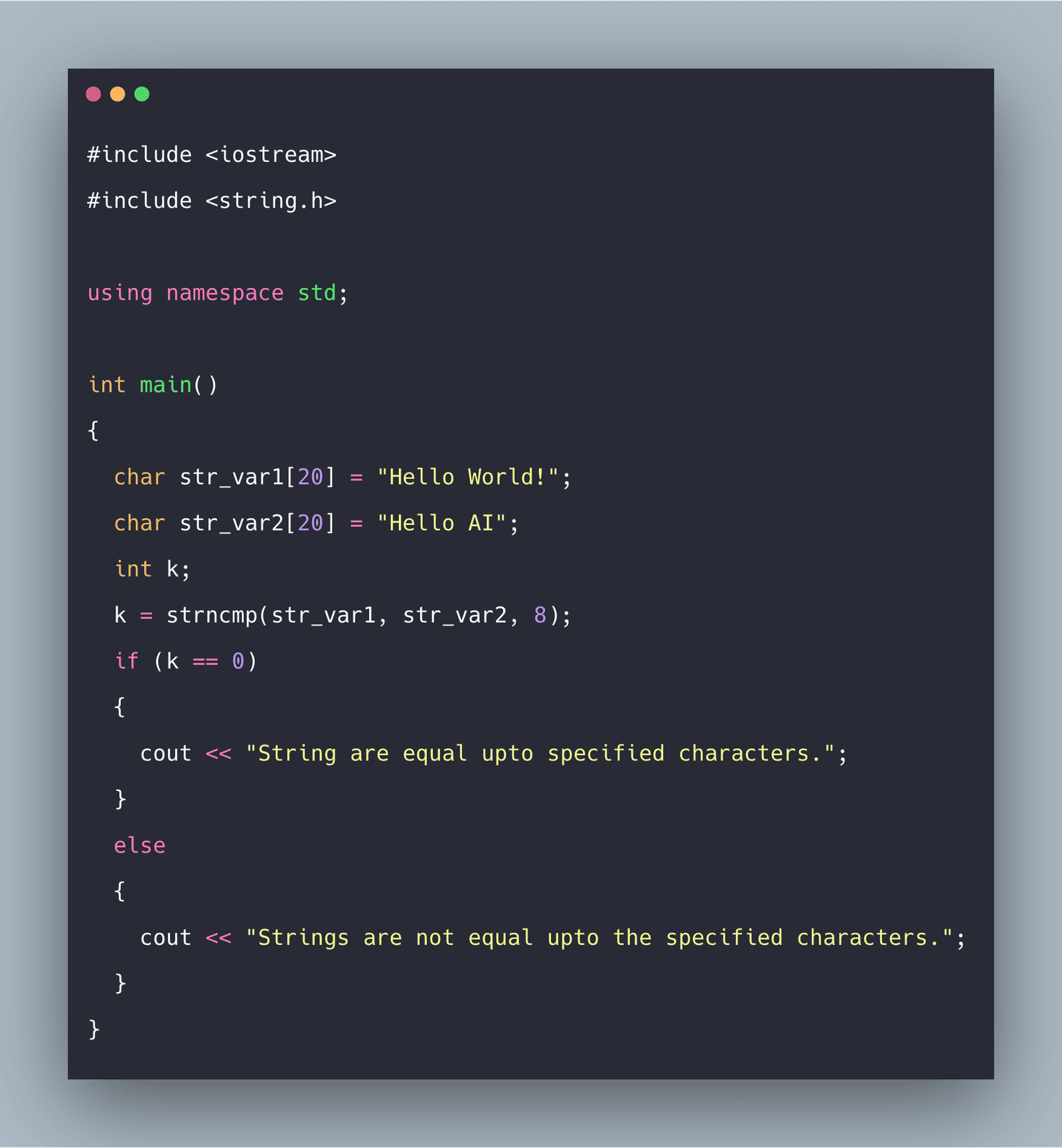# C++ strncmp() Function: The Complete Guide

0
9The std::strncmp() function lexicographically compares not more than count characters from two null-terminated strings and returns the integer based on the outcome.

## C++ strncmp()

The strncmp() function is a built-in string handling function that compares two strings of which null characters terminate the characters. The comparison is done lexicographically and returns an integer. It is defined in string.h header file.

The strncmp() function takes two strings and a number num as arguments and compares at most the first num bytes of both the strings. The num should be equal to the length of the longest string. If the num is defined as greater than a string length, then the comparison is done till the null-character(‘\0’) of either string.

The strncmp() function compares the two strings lexicographically. It starts the comparison from the first character of each string. Then, if. If they are equal to each other, it continues and compares the next character of each string.

This comparison process stops until the terminating null character of either string is reached or the num characters of both the strings match.

### Syntax

```int strncmp(const char* lhs_var, const char* rhs_var, size_t x)
```

Here the function takes three parameters and returns an integer in the main function.

### Parameters

It takes 3 parameters lhs_var, rhs_var, and the count. Then, it compares the characters of lhs_var and rhs_var lexicographically up to the count of the variable “x”.

The function’s behavior is undefined if the string is not ending with null characters.

### Return Value

It returns an integer less than 0 if the first string is less than the second string, equal to zero if the first string is equal to the second string, and greater than 0 if the first string is greater than the second string.

#### Difference between strncmp() and strcmp()

The main difference between strncmp() and strcmp() is that in the case of strncmp(), the comparison is done up to x characters in the string, while in the case of strcmp(), it is done till the strings are terminated by the null character.

### Example programs on strncmp() function in C++

##### Example 1: Write a program to show the working of the strncmp() function.

See the following code.

```#include <iostream>
#include <string.h>

using namespace std;

int main()
{
char str_var1 = "Hello World!";
char str_var2 = "Hello AI";
int k;
k = strncmp(str_var1, str_var2, 8);
if (k == 0)
{
cout << "String are equal upto specified characters.";
}
else
{
cout << "Strings are not equal upto the specified characters.";
}
}```
##### Output
`Strings are not equal up to the specified characters.`
##### Example 2: Write a program to compare two equal strings using strncmp() function.

See the following code.

```#include <iostream>
#include <string.h>

using namespace std;

int main()
{
char str_var1 = "Hello World!";
char str_var2 = "Hello AI";
int k;
k = strncmp(str_var1, str_var2, 5);
if (k == 0)
{
cout << "String are equal upto specified characters.";
}
else
{
cout << "Strings are not equal upto the specified characters.";
}
}```
##### Output
```Strings are equal up to specified characters.
```